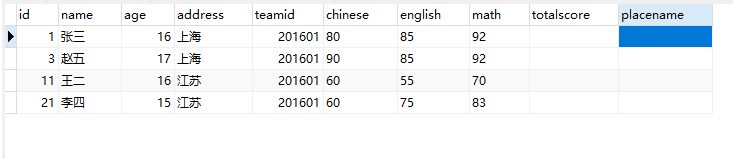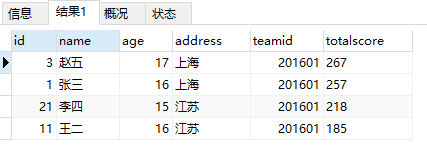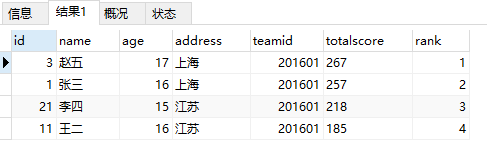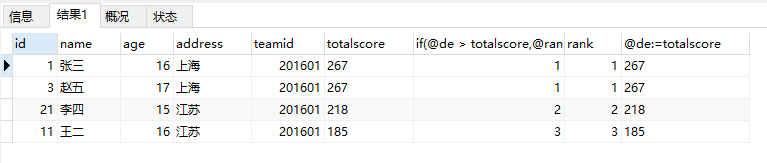# mysql 如何计算数据求和并排出名次

请写出一条sql语句，计算学生表中的‘名次’字段（可以多条sql）select id,name,age,address,teamid,SUM(chinese+math+english)
as totalscore from t_stu group by id order by totalscore descset @rank:=0;
select ta.*,@rank:=@rank+1 as rank from (select id,name,age,address,teamid,SUM(chinese+math+english)
as totalscore from t_stu group by id order by totalscore desc) as taset @de:=0,@rank:=1;
select ta.*,if(@de > totalscore,@rank:=@rank+1,@rank),if(@de = totalscore,@rank:=@rank,@rank) as rank ,@de:=totalscore
from (select id,name,age,address,teamid,SUM(chinese+math+english)
as totalscore from t_stu group by id order by totalscore desc) as ta